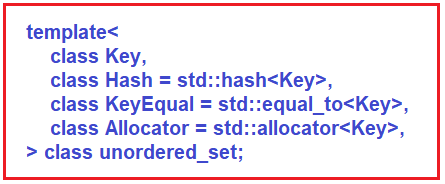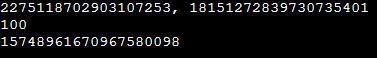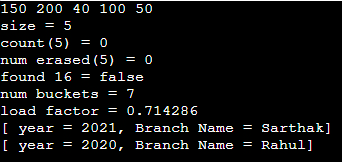# Unordered Set in C++

## Unordered Set in C++ with Examples:

In this article, I am going to discuss Unordered Set in C++ with Examples. Please read our previous article, where we discussed Multiset in C++ with Examples.

##### Unordered Set in C++ with Examples:

We have seen a normal set and multi-set in our previous articles. Both of these were implemented using a balanced binary search tree. So, all the operations were of logarithmic time but here it is constant time because these are implemented using Hash tables. It is an associative container that contains a set of unique elements of the type key. So, this is similar to set which also allows only unique keys and this is different from multi-set which allowed multiple occurrences of the same key.

Here you need to include the <unordered_set> header file. Here everything is constant time. It may not be exactly constant time, that will depend on how good the hash function you have defined is. It is implemented as a hash table.This is the default template. You can see,

1. Key – this is the type of data that we will store.
2. Hash – this is the default std::hash. If you are using an unordered set of some primitive types like in float string then you can use the std::hash you can provide your own hash function. But if you are storing a custom class that you have defined then you will need to provide a custom hash function for this because C++ will not know how to do the hash of objects belonging to that class if you are using primitive types then you do not need to do any of these. So, we will see an example of this.
3. equal_to – this is another key equal function that checks for the equality of keys.
4. allocator – this is the standard allocator.

Now let us see some of the functions of an unordered set.

##### Functions of Unordered Set in C++:
1. size () – it will give the total number of elements in the unordered set.
2. = – the equal operator is used to assign values to it.
3. clear () – it will clear all the elements from the unordered set.
4. count () – it will return either 0 or 1. In the case of a set, it returns 0 or 1 depending on whether the key is present or not. In the case of a multi-set, it will return the actual count of that key.
5. find () – if the key is found it will return the iterator to that and if it is not present in the set, it will return the SET_NAME.end () which is not pointing to any of the elements of the set.
6. empty () – it checks whether there are any elements or not.
7. We have different versions of the insert and erase function which we will see in detail. Some additional functions which we had not seen earlier because these are specific to hash table Implementations and there are many more of these. We have just written a couple of them. These functions you generally do not use but if you are interested you can explore these.
8. bucket_count (), load_factor () – The underlying algorithm is a hash table underlying data structure so it will have bucket count (number of buckets). load factor is how the hash table is loaded.
##### Insert Functions of Unordered Set in C++:

Let us see the insert and erase in detail which is very similar to what we had seen in set and multiset.

1. Insert (Key key): One way to insert is to insert a key so you pass the actual value like we have an unordered set ‘us’ and we want to insert 5 into it. So, we pass the actual key. If it is not present, it will be inserted and then it will return a pair consisting of an iterator to this newly inserted element and also a Boolean value denoting whether insertion took place or not (true or false). If it’s already present, it will not insert again. So, this iterator will again be pointing to 5 only and in this case, it will return false.
2. Insert (iterator pos1, iterator pos2): Next way to insert is you pass two iterator positions. Let us say we have a vector that contains many elements and we want to insert from the x position to the y position from this vector to the unordered set then we will pass this iterator position x beginning of this and y at the end of this. V = {_ _ _ x _ _ _ y _ _ _ _ }. So, y position will actually not be inserted. so, everything before will be inserted into the unordered set. And it does not return anything.
3. Insert (initializer_list l): Another way is to insert using the initializer list. For example, we pass the initializer list {5 10 15 20} and it will insert all the values if they are not present and if they are present, it will not insert that and it also does not return anything.
##### Erase Functions of Unordered Set in C++:
1. erase (iterator pos): If you know the iterator position of a given element, you can pass that and it will erase that element from the set and it will return the iterator following the last element that was removed.
2. erase (iterator pos1, iterator pos2): As we have seen in the insert function, we can also pass two positions in this function so that all the elements between these positions will be removed. So pos1 will be included and pos2 will be excluded. It also returns the iterator following the last element removed.
3. erase (key): If you do not know the position but you know the key you can pass the key and it will remove that key. In the above signatures, we have passed the iterator to the key but here we are passing the key itself. If any key is present in the multiset, it will remove all the elements with the key values. It will return the number of elements removed.
##### Hash Function in C++:
```#include <iostream>
#include <functional>
using namespace std;

int main(){
size_t h1 = hash<string>{}("Hello");
size_t h2 = hash<string>{}("World");
cout << h1 << ", " << h2 << endl;
cout << hash<int>{}(100) << endl;
cout << hash<float>{}(103.3) << endl;
}
```
###### Output:In the above program, we have two variables of size_t that are h1 and h2. You also need to include the functional header file in your program. We have assigned some strings to both h1 and h2. Now if you print the hash values then two different values will be generated. A small change in the string of h1 or in h2 will change the hash value. You can directly generate a hash without using the object. As we have written,

hash<int>{}(100)

It will generate a hash of type int for the number 100. This is how the hash function works.

##### Example to understand Unordered Set in C++:
```#include <iostream>
#include <functional>
#include <unordered_set>

using namespace std;

class Batch{
public:
int year;
string branch_name;

bool operator==(const Batch& b) const{
return (this->year == b.year && this->branch_name == b.branch_name);
}

void print_batch() const {
cout << "[ year = " << year << ", Branch Name = " << branch_name << "]\n";
}

};

class BatchHashFunction{
public:
size_t operator()(const Batch& b) const{
return (hash<int>{}(b.year) + hash<string>{}(b.branch_name));
}
};

int main(){

unordered_set<int> us = {50, 100, 40, 200, 50, 50, 150};
for(int x: us)
cout << x << " ";
cout << endl;

cout << "size = " << us.size() << endl;
cout << "count(5) = " << us.count(5) << endl;
cout << "num erased(5) = " << us.erase(5) << endl;
cout << boolalpha << "found 16 = " << (us.find(16) != us.end()) << endl;
cout << "num buckets = " << us.bucket_count() << endl;